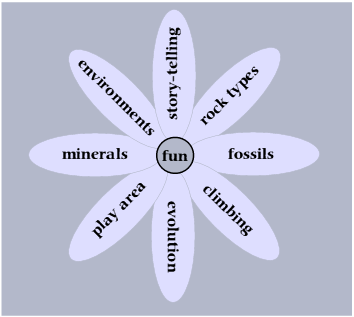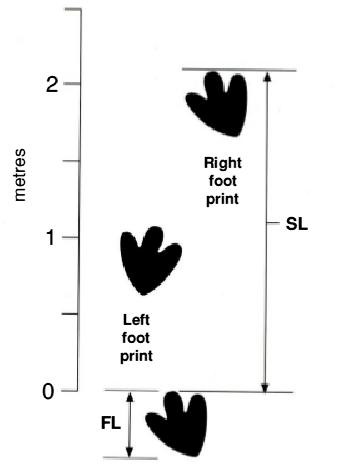Rock Circus Descriptions | Rock Circus Home

Dinosaur footprints

 How to work out the height and gait of the dinosaursDiagram, Pete Loader FL = length of hind foot SL = stride length of either right or left hind foot Equations: h (hip height) = 4 x length of footprint (FL) The relative stride length (SL/h) is used to determine the gait, i.e. whether the animal was: walking (SL/h < 2.0) trotting (SL/h > 2.0 and < 2.9) running (SL/h > or = 2.9) If the foot length of each dinosaur is measured and multiplied by 4, the resulting figure gives the hip height. With this figure, and using the equations provided, it is possible to calculate whether the dinosaur was walking, trotting or running. In the Circus,the arithmetic has been made easy. It was not possible to make the big dinosaur run, as it would have left the circle in two strides! However, the little one walks in and runs out. Either dinosaur could be a carnivore or a herbivore so there are endless possibilities for the stories to be made about them. The layout of the dinosaur trackway and the equations needed to calculate the hip height of the dinosaur and its relative speed. < from the Earthlearningidea activity ‘A dinosaur in the yard’.